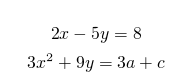## Introduction

The amsmath package provides a handful of options for displaying equations. You can choose the layout that better suits your document, even if the equations are really long, or if you have to include several equations in the same line.

The standard LaTeX tools for equations may lack some flexibility, causing overlapping or even trimming part of the equation when it's too long. We can surpass such difficulties by using the amsmath package, which can be added to preamble of your document using \usepackage{amsmath}.

\begin{equation} \label{eq1}
\begin{split}
A & = \frac{\pi r^2}{2} \\
& = \frac{1}{2} \pi r^2
\end{split}
\end{equation}


The following graphic shows the output produced by the LaTeX code: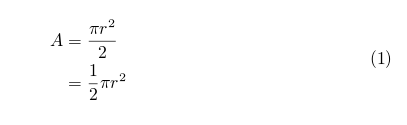You have to wrap your equation in the equation environment if you want it to be numbered, use equation* (with an asterisk) otherwise. Inside the equation environment, use the split environment to split the equations into smaller pieces, these smaller pieces will be aligned accordingly. The double backslash works as a newline character. Use the ampersand character &, to set the points where the equations are vertically aligned.

## Writing a single equation

To display a single equation, as mentioned in the introduction, you have to use the equation* or equation environment, depending on whether you want the equation to be numbered or not. Additionally, you might add a label for future reference within the document.

\begin{equation} \label{eu_eqn}
e^{\pi i} + 1 = 0
\end{equation}

The beautiful equation \ref{eu_eqn} is known as the Euler equation.


The following graphic shows the output produced by the LaTeX code: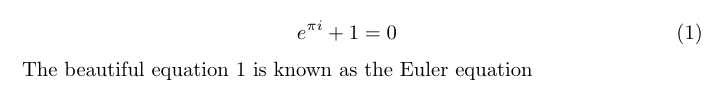## Displaying long equations

For equations longer than a line use the multline environment. Insert a double backslash to set a point for the equation to be broken. The first part will be aligned to the left and the second part will be displayed in the next line and aligned to the right.

Again, the use of an asterisk * in the environment name determines whether the equation is numbered or not.

\begin{multline*}
p(x) = 3x^6 + 14x^5y + 590x^4y^2 + 19x^3y^3\\
- 12x^2y^4 - 12xy^5 + 2y^6 - a^3b^3
\end{multline*}


The following graphic shows the output produced by the LaTeX code: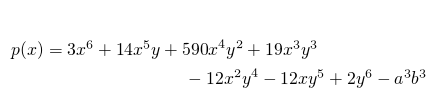## Splitting and aligning an equation

Split is very similar to multline. Use the split environment to break an equation and to align it in columns, just as if the parts of the equation were in a table. This environment must be used inside an equation environment. For an example check the introduction of this document.

## Aligning several equations

If there are several equations that you need to align vertically, the align environment will do it:

\begin{align*}
2x - 5y &=  8 \\
3x + 9y &=  -12
\end{align*}


The following graphic shows the output produced by the LaTeX code: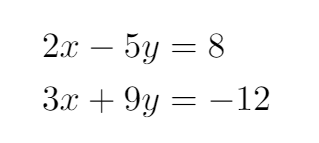Usually the binary operators (>, < and =) are the ones aligned for a nice-looking document.

As mentioned before, the ampersand character & determines where the equations align. Let's check a more complex example:

\begin{align*}
x&=y           &  w &=z              &  a&=b+c\\
2x&=-y         &  3w&=\frac{1}{2}z   &  a&=b\\
-4 + 5x&=2+y   &  w+2&=-1+w          &  ab&=cb
\end{align*}


The following graphic shows the output produced by the LaTeX code: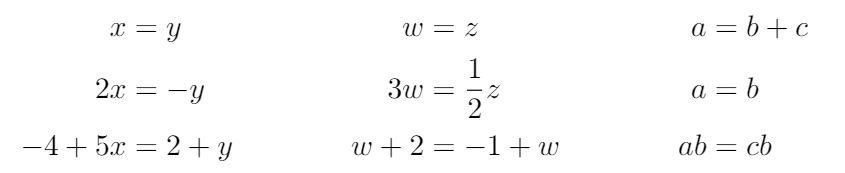Here we arrange the equations in three columns. LaTeX assumes that each equation consists of two parts separated by an & and that each equation is separated from the one before by an &.

Again, use * to toggle the equation numbering. When numbering is allowed, you can label each row individually.

## Grouping and centering equations

If you just need to display a set of consecutive equations, centered and with no alignment whatsoever, use the gather environment. The asterisk trick to set/unset the numbering of equations also works here.

\begin{gather*}
2x - 5y =  8 \\
3x^2 + 9y =  3a + c
\end{gather*}


The following graphic shows the output produced by the LaTeX code: